# Test: Stoichiometry Level - 2

## 40 Questions MCQ Test Chemistry Class 11 | Test: Stoichiometry Level - 2

Description
This mock test of Test: Stoichiometry Level - 2 for Class 11 helps you for every Class 11 entrance exam. This contains 40 Multiple Choice Questions for Class 11 Test: Stoichiometry Level - 2 (mcq) to study with solutions a complete question bank. The solved questions answers in this Test: Stoichiometry Level - 2 quiz give you a good mix of easy questions and tough questions. Class 11 students definitely take this Test: Stoichiometry Level - 2 exercise for a better result in the exam. You can find other Test: Stoichiometry Level - 2 extra questions, long questions & short questions for Class 11 on EduRev as well by searching above.
QUESTION: 1

### For the reaction         2x + 3y + 4z → 5w Initially if 1 mole of x, 3 mole of y and 4 mole of z is taken. If 1.25 mole of w is obtained then % yield of this reaction is

Solution:

2x + 3y + 4z     =      5w
Moles                        1      3       4
Stoichiometric coff.    2      1       1
Ratio                         0.5    1        1
So, x is Limiting reagent.
So 0.5 = moles of w/5  (theoritical)
Or moles of w = 2.5
But obtained moles= 1.25
Therefore % yield = 1.25/2.5* 100 = 50%

QUESTION: 2

### A solution of A (MM = 20 ) and B (MM =10), [Mole fraction XB = 0.6] having density 0.7 gm/ml then molarity and molality of B in this solution will be _____ and _____ respectively.

Solution:

The correct answer is Option A.

Given, molar mass of A, MA = 20g/mol.
and molar mass of B, MB = 10g/mol.
density of solution , d = 0.7 g/ml
mole fraction of B, xB = 0.6
so, mole fraction of A , xA = 1 - 0.6 = 0.4
using formula,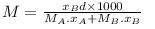= 0.6 × 0.7 × 1000/(20 × 0.4 + 10 × 0.6)
= 420/14
= 30 molar
Hence, molarity of the solution is 30M
use formula,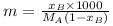where m is the molality of the solution.
so, m = 600/(20 × 0.4)
= 600/8
= 75 molal
Hence, molality of the solution is 75m

QUESTION: 3

### 125ml of 8% w/w NaOH solution (sp. gravity 1) is added to 125 ml of 10% w/v HCl solution. The nature of resultant solution would be _____

Solution:

In 125 ml of 10% NaOH solution.
The mass of NaOH will be,
Mass% = (Mass of NaOH/Volume of solution)*100
= 10% = (Mass of NaOH/125)*100
Mass of NaOH = 12.5g
Mass of NaOH = 12.5/40
= 0.3125 mole
In 125 ml of 10% HCl solution.
The mass of HCl will be,
Mass% = (Mass of HCl/Volume of solution)*100
= 10% = (Mass of HCl/125)*100
Mass of NaOH = 12.5g
Mass of NaOH = 12.5/36.5
= 0.3424 mole
The balanced chemical reaction will be,
NaOH + HCl ----> NaCl + H2O
From balanced reaction we conclude that,
As, 1 mole of NaOH will neutralize 1 mole of HCl
So, 0.3125 moles will neutralize 0.3125 moles of HCl
Thus, the moles of HCl left un-neutralized = 0.3424 - 0.3125 = 0.0299 mole
That means, the moles of acid are still present in the solution after mixing. So, the solution will become acidic is nature.

QUESTION: 4

Ratio of masses of H2SO4 and Al2(SO4)3 is grams each containing 32 grams of S is _____

Solution:

Molar mass of the Aluminium sulphate = 342 g/mole.
Mass of the sulphur present in 1 mole of the compound = 32 × 3 = 96 g.
Since, 96 g of S is present in 342 g of compound.
Thus, 32 g of S is present in 114 g of the compound.
Thus, the mass of the Aluminium sulphate is 114 g.
Now,
Molar mass of Sulphuric Acid = 98 g/mole.
Mass of sulphur in 1 mole = 32 g.
Since, 32 g of S is present in 98 g of Acid.
Thus, 32 g of the S is present in 98 g of the Acid.
Now, for the ratio,
Mass of Acid/Mass of Aluminium Sulphate = 98/114
= 49/54 = 0.86

QUESTION: 5

The vapour density of a mixture of gas A (Molecular mass = 40) and gas B (Molecular mass = 80) is 25. Then mole % of gas B in the mixture would be

Solution: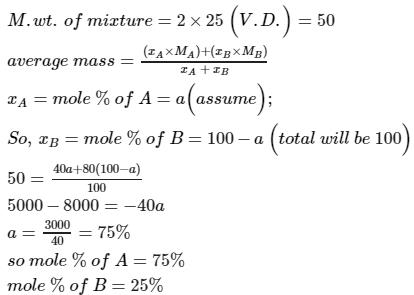QUESTION: 6

For the reaction                    2A + 3B + 5C → 3D

Initially if 2 mole of A, 4 mole of B and 6 mole of C is taken, with 25% yield, moles of D which can be produced are _________

Solution:

2A + 3B + 5C → 3D
2    4     6
From given data, it can be seen that A is the limiting reagent.
2 mol of A will produce 3 mol of D. As yield of reaction is 25% only so, mol of D produced
= 3×0.25
= 0.75 mol.

QUESTION: 7

Two elements X (atomic mass = 75) and Y (atomic mass = 16) combine to give a compound having 75.8% of X. The formula of the compound is :

Solution:

Let the total mass be 100 gm.
This includes 75.8 gm of X and 24.2 gm of Y
Moles of X = 75.8/75 ∼ 1
Moles of Y = 24.2/16 = 3/2
Therefore, X:Y = 1:3/2 = 2:3
So the formula is X2Y3.

QUESTION: 8

Equal volumes of 10% (v/v) of HCl is mixed with 10% (v/v) NaOH solution. If density of pure NaOH is 1.5 times that of pure HCl then the resultant solution be :

Solution:

Since the density of pure NaOH is 1.5 times that of pure HCl and the volumes (and volume percent) are equal, the mass (and moles) of NaOH will be 1.5 times that of HCl.
NaOH is in excess.
Therefore, the resultant solution will be basic.

QUESTION: 9

10 ml of a compound containing 'N' & 'O' is mixed with 30 ml of H2 to produce H20(l) and 10 ml of N2(g). Molecular formula of compound if both reactants react completely, is

Solution:

At STP 1 mole of any gas occupies 22.4 L. Hence, the volume of the gases can be correlated to the number of moles of gases. 10 ml of compound reacts with 30 ml of hydrogen to produce water and 10 ml of nitrogen. Thus, for every one mole of the compound, 3 moles of hydrogen reacts to produce water and 1 mole of nitrogen.

X+3H2→H2O+N2
This equation will be balanced when the formula of the compound is ,

N2O3, N2O3+3H2→3H2O+N2

QUESTION: 10

The percentage by mole of NO2 in a mixture NO2(g) and NO (g) having average molecular mass 34 is :

Solution: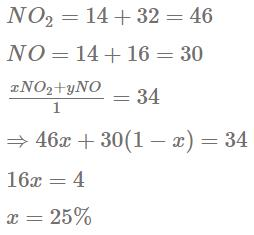QUESTION: 11

Assuming complete precipitation of AgCl, calculate the sum of the molar concentration of all the ions if 2 lit of 2M Ag2SO4 is mixed with 4 lit of 1 M NaCl solution is :

Solution:

Moles of Ag2 SO4 = 2M x 2L = 4 mol
Moles of NaCl = 1M x 4L = 4 mol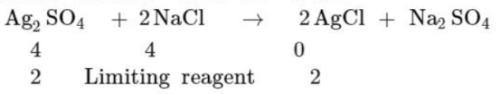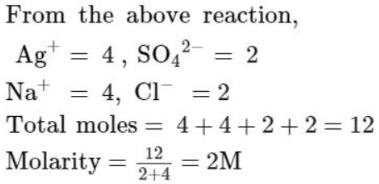QUESTION: 12

What volumes should you mix of 0.2M NaCl and 0.1M CaClsolution soo that in resulting solution the concentration of positive ion is 40% lesser than concentration of negative ion. Assuming total volume of solution 1000ml.

Solution:

Molarity of NaCl, M1 = 0.2 M
Molarity of CaCl2, M2 = 0.1 M
Total Volume of mixture, V = 1000 mL
Let volume of NaCl, V1 = x
Volume of CaCl2 = 1000-x
Since concentration  of cation is 40% less than anion, for every 100 anions contain 60 cation.
NaCl → Na+  +  Cl-
CaCl2 → Ca2+ + 2Cl-
Moles of cation, n1 = 1×M1V  + 1×M2V2
= 0.2 x x + 0.1(1000-x)
= (0.1x + 100) moles
Moles of anion, n2 = 1×M1V1 + 2×M2V2
= 1×0.2 x x + 2×0.1(1000-x)
200 moles
According to question,
n2-n1/n2 × 100 = 40%
200-(0.1x +100)/200 × 100 = 40
100 - 0.1x = 80
x = 200
Therefore, volume of NaCl, V1 = 200 mL
Volume of CaCl2, V2 = (1000-200) = 800 mL

QUESTION: 13

Weight of oxygen in Fe2O3 and FeO is in the simple ratio for the same amount of iron is :

Solution:

Molar mass of Fe = 56 gm
Molar mass of O = 16 gm
In Fe2O3, mass of Fe = 56 × 2 = 112
Mass of O in Fe2O3 = 48
In FeO, mass of Fe = 56. Since we need to have the same mass of Fe, we need to  take 2 moles of FeO.
Mass of O in 2 moles of FeO = 16 2 =32
Therefore, ratio of mass of O in Fe2O3 and FeO = 48/32 = 3:2

QUESTION: 14

The number of atoms present in 0.5 g-atoms of nitrogen is same as the atoms in

Solution:

Atomic mass of nitrogen = 14 g

Therefore, mass of 1 mole of nitrogen = 14g

Now, 1 mole = 6.022 x 1023 atoms

Since, 1 g-atom = 1 mole

0.5 g-atom = 0.5 moles

Therefore, no. of atoms in 0.5 moles of Nitrogen = 6.022 x 1023 x 0.5

= 3.011 x 1023

Now,

12 g carbon = 1 mole carbon

32 g sulphur = 1 mole sulphur

8 g oxygen = 0.5 mole oxygen

24 g magnesium = 1 mole magnesium

Number of atoms in 0.5 mole oxygen = = 3.011 x 1023

QUESTION: 15

The oxide of a metal contains 30% oxygen by weight. If the atomic ratio of metal and oxygen is 2 : 3, determine the atomic weight of metal.

Solution:

From the question, formula of oxide = M2O3
%age of oxygen = mass of oxygen in molecule/mass of molecule = 30/100
(16×3)/(2 M+16×3) = 30/100
M = 160-48/2 = 56

QUESTION: 16

The O18/O16 ratio in some meteorites is greater than that used to calculate the average atomic mass of oxygen on earth. The average mass of an atom of oxygen in these meteorites is _____ that of a terrestrial oxygen atom?

Solution:

Average atomic mass of an element is defined as the summation of the product of the atomic masses and fractional abundances of its isotopes.
Equation to calculate the average atomic mass will be given by:
∑_(i=1 n (Atomic mass of an isotope)i × (fractional abundance)
We are given that the abundance ratio of the oxygen in meteorites is more than the terrestrial oxygen atom.
As, from the formula, average atomic mass of an element is directly proportional to the fractional abundance of the isotopes. Hence, with increase in the abundance, the average atomic mass also increases.
Therefore, the average mass of the oxygen atom in these meteorites will be greater than the terrestrial oxygen atom.

QUESTION: 17

Mass of one atom of the element A is 3.9854 x 10-23 . How many atoms are contained in 1g of the element A?

Solution:

In any element or substance, the number of atoms contained in 1 mole is 6.023 × 10²³ atoms. This is Avogadro's number and is constant in 1 mole of every substance.

mass of 1 atom = mass of a mole of atoms / 6.022 x 10²³

That is molar mass = Avogadro's number × mass of 1 atom

molar mass for A = 6.022 x 10²³ × 3.9854×10⁻²³
= 24

Molar mass of element A is 25g/mol

Find number of atoms in 1 gram of element:

If molar mass is 24 g/mol means 1 mole has 24g.

If 24g = 1 mole = 6.022 x 10²³ atoms

Then 1g = 6.022 x 10²³ × 1g/24g

= 2.5091667 ×10²² atoms

Therefore  1g of element A has 2.5091667 ×10²² atoms

QUESTION: 18

Which has the maximum number of atoms of oxygen

Solution:

Actually 1 mole of H2O contains Avogadro number of H2O molecules. But since each H2O molecule has only 1 Oxygen atom, we say that
number of H2O molecules = number of oxygen atoms
We may also say that the number of Oxygen atoms is directly proportional to the product of number of moles and number of Oxygen atoms in the molecule.
(a) 1 mole of H2O has 22,400 mL
Moles of H2O in 10 mL = 10/22400 = 4.46 10 -4
Moles of O = 4.46×10-4
(b) moles of O = 0.1 x 5 = 0.5
(c) number of moles of O3 = 12/48 = 0.25
No of moles of O =  0.25 x 3 = 0.75
(d) 6 x 1023 molecules →1 mole
12 x 1022 molecules → 0.2 mole
Moles of O = 0.2 x 2 = 0.4
Therefore the maximum number of oxygen atoms are present in 0.1 moles of √V2O5

QUESTION: 19

The density of quartz mineral was determined by adding a weighed piece to a graduated cylinder containing 51.2ml water. After the quartz was submersed, the water level was 65.7 ml. The quartz piece weighed  38.4g. What was the density of quartz?

Solution:

Density=mass/volume.
Therefore density of quartz =mass /change in volume of water
Change in volume gives volume of quartz.
Density=38.4/65.7-51.2
=38.4/14.5
=2.648~2.65

QUESTION: 20

A sample of clay contains 40% sillica and 15% water. The sample is partially dried by which it loses 5 gm water. If the percentage of water in the partially dried clay is 8, calculate the percentage of silica in the partially dried clay.

Solution:

Clay original sample contains 40% silica , 15% water so total non water component is 100 - 15 = 85 gram .Now sample is partially dried and loses 5 gram water .Again dried clay contains 8 % water means 8 g water . Therefore total non water component is 100-8 = 92 gram.Now percent of silica in partially dried sample will be 40/85×92= 43.29%

QUESTION: 21

A definite amount of gaseous hydrocarbon was burnt with just sufficient amount of O2. The volume of all reactants was 600 ml, after the explosion the volume of the products [CO2(g) and H2O(g)] was found to be 700 ml under the similar conditions. The molecular formula of the compound is :

Solution:

Let the molecular formula of the compound be CmHn.
600 ml of reactants after reaction gives 700 ml of products.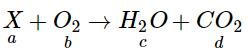The number of moles are directly proportional to the volume of the gas.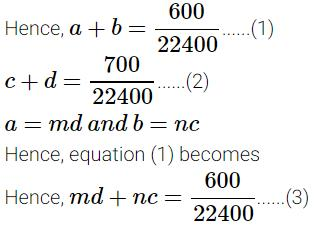When equations (2) and (3) are solved, m=3 and n=8
Hence, the molecular formula of the compound is C3H8.

QUESTION: 22

One gram is silver salt of an organic dibasic acid yields , on strong heating , 0.5934 g of silver. if the weight percentage of carbon in it 8 times the weight percentage of hydrogen and half the weight percentage of oxygen, determine the molecular formula of the acid. [Atomic weight of Ag = 108]

Solution:

H2A → Ag2A → Ag
Mass of compound = 1 gm
Mass of Ag in compound = 0.5934 gm
Since same mole of Ag be on both sides,
2 /216+MA = 0.5934/108
Or MA = 148
Therefore molar mass of acid(H2A) = 148+2 = 150gm
Let %age of C = x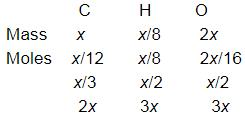Therefore, empirical formula = C2H3O3
(Empirical mass) n = molar mass
n = 150/75 =2
Therefore, molecular formula = C4H6O6

QUESTION: 23

C6H5OH(g) + O2(g) → CO2(g) + H2O(/)
Magnitude of volume change if 30 ml of C6H5OH (g) is burnt with excess amount of oxygen, is

Solution:

The reaction is not balanced. The balanced reaction is
C6H5OH (g) + 7O2(g)  →  6CO2(g) + 3H2O(l)
Sinec water formed in liquid state, imts volume is considered to be 0.
Volume of C6H5OH = 30 mL
Since 1 volume of C6H5OH reacts with 7 volume of O2,
Volume of O2 = 7×30 = 210mL
7 moles of O2 forms 6 moles of CO2
1 moles of O2 forms 6/7 volume of CO2
210 mL forms 6/7 210 = 180mL
Volume change = (total volume)reactant - (total volume)product
= (30+210)-(180+0) = 60 mL

QUESTION: 24

Similar of % labelling of oleum, a mixture of H3PO4 and P4O10 is labelling as ( 100 + x ) % where x  is the maximum mass of water which can react with P4O10 present in 100 gm mixture of H3PO4 and P4O10. If such a mixture is labelled as 127% Mass of P4O10 is 100 gm of mixture, is

Solution:

P4O10 + 6 H2O --------> 4 H3PO4

(284 gm + 6*18               = 108 gm)

mass of P4O10 = (127 – 100)*284 / 108 = 71 gm

QUESTION: 25

Mass of sucrose C12H22O11 produced by mixing 84 gm of carbon, 12 gm of hydrogen and 56 lit.O2 at 1 atm & 273 K according to given reaction, is C(s) + H 2(g) + O2(g) → C12H22O11(s)

Solution: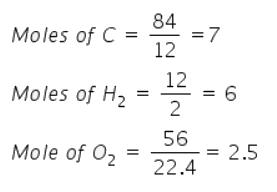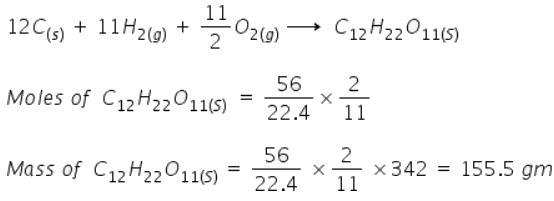QUESTION: 26

If 50 gm oleum sample rated as 118% is mixed with 18 gm water, then the correct option is

Solution:

18 % ⇒ 100 g sample uses 18 g water
⇒ 50 g sample need 9 g water
Since we have added 18 gm water. But according to the question, only 9 gm of water is needed.
⇒ So we have 59 g H2SO4 + 9 g water

QUESTION: 27

In the quantitative determination of nitrogen using Duma’s method, N2 gas liberated from 0.42 gm of a sample of organic compound was collected over water. If the volume of N2 gas collected was 100/11 ml at total pressure 860 mm Hg at 250 K, % by mass of nitrogen in the organic compound is
[Aq. tension at 250K is 24 mm Hg and R = 0.08 L atm mol–1 K–1]

Solution:

The volume of dry nitrogen at STP is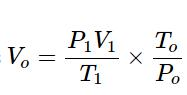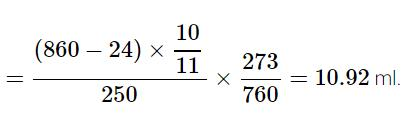Percentage by mass of nitrogen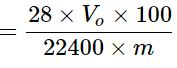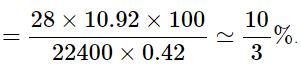QUESTION: 28

40 gm of a carbonate of an alkali metal or alkaline earth metal containing some inert impurities was made to react with excess HCl solution. The liberated CO2 occupied 12.315 lit. at 1 atm & 300 K. The correct option is

Solution:

To calculate the volume of CO2 at STP condition,
Let say it will be V1
1×V / 273 = 1×12.315 / 300
​⇒V1=11.2L at STP
11.2L of CO2 at STP is equivalent to 0.5 mole of CO2. Suppose, Li is the metal
∴Li2CO3+2HCl⟶2LiCl+H2O+CO2
​∴  0.5 mole of Li2CO3 corresponds to 0.5×73.89 i.e. 37gm of Li2CO3
​∴  Wt. of impurity will be (40−37)gm i.e. 3gm
= 36.5×1 / 0.73=50

QUESTION: 29

The minimum mass of mixture of A2 and B4 required to produce at least 1 kg of each product is:
(Given At. mass of ‘A’ = 10; At mass of ‘B’ = 120) 5A2 + 2B4 → 2AB2 + 4A2B

Solution:

Molar masses of AB2 and A2B are 250 g/mol and 140 g/mole respectively.
1 kg of AB2 corresponds to 4 moles. It requires 10 mole A2 and 4 mole B4 respectively.
1 kg of A2B corresponds to 7.14 mole. It requires 8.9 mole A2 and 3.57 mole B4 respectively.
Thus 10 mole A2 (200 g) and 4 mole B4 (1920  g) will produce at least 1 kg of each product.
The minimum mass of mixture required is 200g+1920g=2120g.

QUESTION: 30

The mass of CO2 produced from 620 gm mixture of C2H4O2 & O2, prepared to produce maximum energy is

Solution:

We know when a fuel undergoes complete combustion it produces carbon dioxide and water.

The combustion reaction is: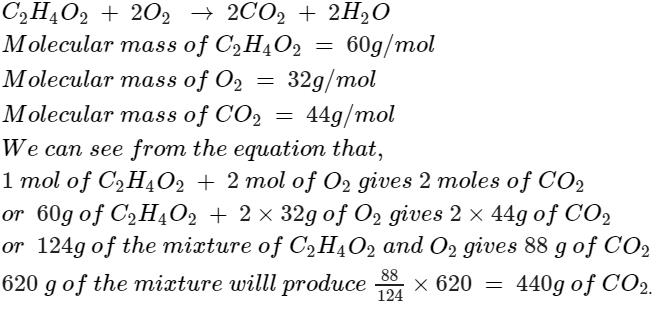QUESTION: 31

12.5 gm of fuming H2SO4 (labelled as 112%) is mixed with 100 lit water. Molar concentration of H+ in resultant solution is :
[Note : Assume that H2SO4 dissociate completely and there is no change in volume on mixing]

Solution:

The correct answer is Option A.

Given :
mass = 12.5 gm
% of H2SO4 = 112 %
= 112 /100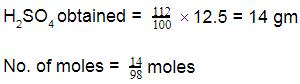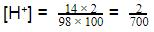QUESTION: 32

74 gm of sample on complete combustion gives 132 gm CO2 and 54 gm of H2O. The molecular formula of the compound may be

Solution:

Mass of C in 132g CO2 = 12/44*132 = 36g

Mass of H in 54g H2O = 2/18*54 = 6g

Missing mass is O = 74g - ( 36+6) = 32g

Divide each mass by atomic mass :

C = 36/12 = 3

H = 6/1 = 6

O = 32/16 = 2

QUESTION: 33

The % by volume of C4H10 in a gaseous mixture of C4H10, CH4 and CO is 40. When 200 ml of the mixture is burnt in excess of O2. Find volume (in ml) of CO2 produced.

Solution:

There is one Carbon atom in the molecule of CO2.

Volume of C4 H10  in the mixture = 80 ml

volume of CO2 produced by combustion of C4H10 = 4 * 80 = 320 ml

as there are 4 Carbons in each molecule.

volume of CH4 + CO in mixture = 120 ml

volume of CO2 produced = 120 ml

Total volume of CO2 = 320 + 120 = 440 ml

QUESTION: 34

An iodized salt contains 0.5% of Nal. A person consumes 3 gm of salt everyday. The number of iodide ions going into his body everyday is

Solution: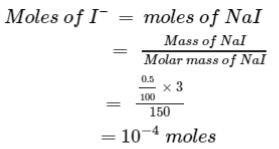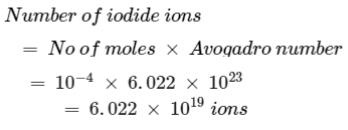QUESTION: 35

The pair of species having same percentage (mass) of carbon is :

Solution:

Percentage of carbon in acetic acid = 24/60×100 =40%
Percentage of carbon in C6H12O6 = 72/180×100 = 40%

QUESTION: 36

200 ml of a gaseous mixture containing CO, CO2 and N2 on complete combustion in just sufficient amount of O2 showed contraction of 40 ml. When the resulting gases were passed through KOH solution it reduces by 50 % then calculate the volume ratio of VCO2 :VCO : VN2 in original mixture.

Solution:

Volume of mixture of CO, CO2 and N2 = 200 mL
Volume of CO = x mL
Volume of N2 = y mL
Volume of CO2 = 200 - x  - y mL
On combustion, CO2 remains as it is.  Nitrogen burns only at very
high temperatures. At low temperatures it does not form oxides.
2CO  +  O2  →  2 CO2
x mL x/2 mL
x mL
In the input the amount of O2 present = x/2 mL
Total volume of gas mixture + O2 = 200 + x/2 ml
Resulting mixture: total : 200 ml as:
N4: y ml  CO2:  x + (200 - x - y) = 200 - y  ml
Contraction (reduction) in volume of gases is
40 ml = 200 + x/2 - 200 = x/2
x = volume of CO in the mixture = 80 ml
Now the mixture of CO2 + N2 is passed through base KOH. All CO2 reacts and N2
is not reactive with   KOH.
2 KOH + CO2 → K2CO3 + H2O
So volume of gas mixture reduces by 50% of 200 ml ie., 100 ml.
⇒ 200 - y = 100 ml
⇒ y = 100 ml
So we had 80 ml of CO, 100 ml of N2, and 20 ml of CO2 in the mixture initially.
Ratio: of volumes of  CO2 : CO : N2  =  1 : 4 : 5

QUESTION: 37

Density of a gas relative to air is 1.17. Find the mol. mass of the gas. [Mair = 29 g/mol]

Solution:

Mol mass of gas = relative density of gss  * mol. Mass of air = 1.17 * 29 = 33.9

QUESTION: 38

Two elements X (atomic mass 16) and Y (atomic mass 14) combine to form compounds A, B and C. The ratio of different masses of Y which combines with a fixed mass of X in A, B and C is 1:3:5. If 32 parts by mass of X combines with 84 parts by mass of Y in B, then in C, 16 parts by mass of X will combine with___ parts by mass of Y.

Solution:

In B, 32 parts of x combine with y=84 parts
16 parts of x will combine with y =42parts
Now, the number of parts of x in both B and C is of equal masses of y which combine with a fixed mass of x in B and C in the ratio 3:5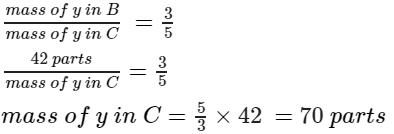QUESTION: 39

In a textile mill, a double-effect evaporator system concentrates weak liquor containing 4% (by weight) caustic soda to produce a dye containing 25% solids (by weight). Calculate the weight of the water evaporate per 100-kg feed in the evaporator.

Solution:

basis: 100 kg weak liquor(feed)
It contains 4 kg caustic soda.Let quantity of Iye be z kg.
Caustic soda in Iye = .25kg z
However caustic soda does not take part in the evaporation.
.25z =4
z=4/.25
= 16 kg
Evaporation = 100–16 kg = 84 kg

QUESTION: 40

Zinc ore (zinc sulphide) is treated with sulphuric acid, leaving a solution with some undissolved bits of material and releasing hydrogen sulphide gas. If 10.8g of zinc ore is treated with 50.0 ml of sulphuric acid (density 1.153 g/ml), 65.1g of solution and undissolved material remains. In addition, hydrogen sulphide (density 1.393 g/L) is evolved. What is the volume (in liters) of this gas?

Solution: